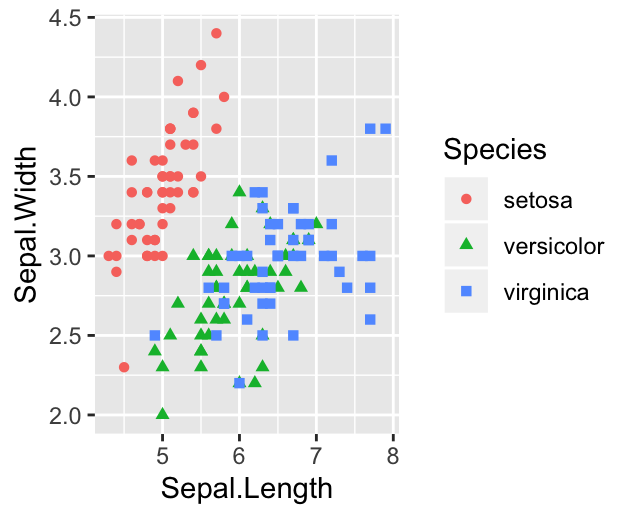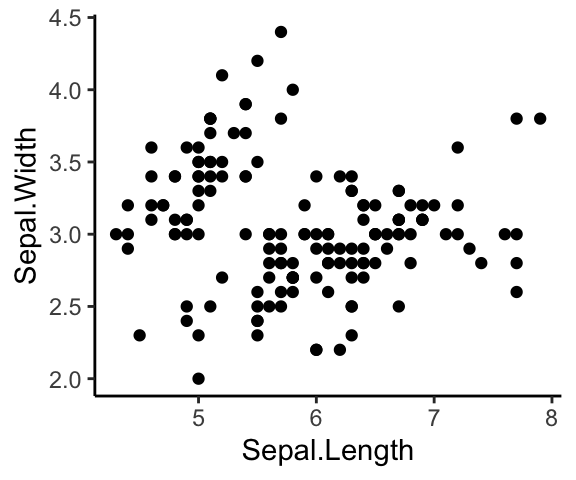# Data Visualization using GGPlot2

## What is ggplot2

GGPlot2 is a powerful and a flexible R package, implemented by Hadley Wickham, for producing elegant graphics piece by piece (Wickham et al. 2017).

The gg in ggplot2 means Grammar of Graphics, a graphic concept which describes plots by using a “grammar”. According to the ggplot2 concept, a plot can be divided into different fundamental parts: Plot = data + Aesthetics + Geometry

• data: a data frame
• aesthetics: used to indicate the x and y variables. It can be also used to control the color, the size and the shape of points, etc…..
• geometry: corresponds to the type of graphics (histogram, box plot, line plot, ….)

The ggplot2 syntax might seem opaque for beginners, but once you understand the basics, you can create and customize any kind of plots you want.

Note that, to reduce this opacity, we recently created an R package, named ggpubr (ggplot2 Based Publication Ready Plots), for making ggplot simpler for students and researchers with non-advanced programming backgrounds.

Contents:

#### Related Book

GGPlot2 Essentials for Great Data Visualization in R

## Key functions

After installing and loading the ggplot2 package, you can use the following key functions:

Plot types GGPlot2 functions
Initialize a ggplot ggplot()
Scatter plot geom_point()
Box plot geom_boxplot()
Violin plot geom_violin()
strip chart geom_jitter()
Dot plot geom_dotplot()
Bar chart geom_bar() or geom_col()
Line plot geom_line()
Histogram geom_histogram()
Density plot geom_density()
Error bars geom_errorbar()
QQ plot stat_qq()
ECDF plot stat_ecdf()
Title and axis labels labs()

## Example of plots

The main function in the ggplot2 package is ggplot(), which can be used to initialize the plotting system with data and x/y variables.

For example, the following R code takes the iris data set to initialize the ggplot and then a layer (geom_point()) is added onto the ggplot to create a scatter plot of x = Sepal.Length by y = Sepal.Width:

library(ggplot2)
ggplot(iris, aes(x = Sepal.Length, y = Sepal.Width))+
geom_point()

# Change point size, color and shape
ggplot(iris, aes(x = Sepal.Length, y = Sepal.Width))+
geom_point(size = 1.2, color = "steelblue", shape = 21)Note that, in the code above, the shape of points is specified as number. The different point shape available in R, include:It’s also possible to control points shape and color by a grouping variable (here, Species). For example, in the code below, we map points color and shape to the Species grouping variable.

Note that, a ggplot can be holded in a variable, say p, to be printed later

# Control points color by groups
ggplot(iris, aes(x = Sepal.Length, y = Sepal.Width))+
geom_point(aes(color = Species, shape = Species))

# Change the default color manually.
# Use the scale_color_manual() function
p <- ggplot(iris, aes(x = Sepal.Length, y = Sepal.Width))+
geom_point(aes(color = Species, shape = Species))+
scale_color_manual(values = c("#00AFBB", "#E7B800", "#FC4E07"))
print(p)## Legend position

The default legend position is “right”. Use the function theme() with the argument legend.position to specify the legend position.

Allowed values for the legend position include: “left”, “top”, “right”, “bottom”, “none”.

Examples:

# Change legend position to the top
p + theme(legend.position = "top")To remove legend, use p + theme(legend.position = “none”).

## Titles and axis labels

The function labs() can be used to change easily the main title, the subtitle, the axis labels and captions.

p + labs(
title = "Edgar Anderson's Iris Data",
subtitle = "iris is a data frame with 150 cases (rows) and 5 variables",
x = "Sepal Length (cm)", y = "Sepal Width (cm)"
)## Facet: Plot with multiple pnels

You can also split the plot into multiple panels according to a grouping variable. R function: facet_wrap(). Another interesting feature of ggplot2, is the possibility to combine multiple layers on the same plot. For example, with the following R code, we’ll:

• Add points with geom_point(), colored by groups.
• Add the fitted smoothed regression line using geom_smooth(). By default the function geom_smooth() add the regression line and the confidence area. You can control the line color and confidence area fill color by groups.
• Facet the plot into multiple panels by groups
• Change color and fill manually using the function scale_color_manual() and scale_fill_manual()
ggplot(iris, aes(x = Sepal.Length, y = Sepal.Width))+
geom_point(aes(color = Species))+
geom_smooth(aes(color = Species, fill = Species))+
facet_wrap(~Species, ncol = 3, nrow = 1)+
scale_color_manual(values = c("#00AFBB", "#E7B800", "#FC4E07"))+
scale_fill_manual(values = c("#00AFBB", "#E7B800", "#FC4E07"))## GGPlot theme

Note that, the default theme of ggplots is theme_gray() (or theme_grey()), which is theme with grey background and white grid lines. More themes are available for professional presentations or publications. These include: theme_bw(), theme_classic() and theme_minimal().

To change the theme of a given ggplot (p), use this: p + theme_classic(). To change the default theme to theme_classic() for all the future ggplots during your entire R session, type the following R code:

theme_set(
theme_classic()
)

Now you can create ggplots with theme_classic() as default theme:

ggplot(iris, aes(x = Sepal.Length, y = Sepal.Width))+
geom_point()## Further customizations of a ggplot

You can read more in our online ggplot cheatsheet, GGPlot Cheat Sheet for Great Customization, which describes how to:

• Add title, subtitle, caption and change axis labels
• Change the appearance - color, size and face - of titles
• Set the axis limits
• Set a logarithmic axis scale
• Rotate axis text labels
• Change the legend title and position, as well, as the color and the size
• Change a ggplot theme and modify the background color
• Add a background image to a ggplot
• Use different color palettes: custom color palettes, color-blind friendly palettes, RColorBrewer palettes, viridis color palettes and scientific journal color palettes.
• Change point shapes (plotting symbols) and line types
• Rotate a ggplot
• Annotate a ggplot by adding straight lines, arrows, rectangles and text.

## Save ggplots

You can export a ggplot to many file formats, including: PDF, SVG vector files, PNG, TIFF, JPEG, etc.

The standard procedure to save any graphics from R is as follow:

1. Open a graphic device using one of the following functions:
• pdf(“r-graphics.pdf”),
• svg(“r-graphics.svg”),
• png(“r-graphics.png”),
• tiff(“r-graphics.tiff”),
• jpeg(“r-graphics.jpg”),
• and so on.

Additional arguments indicating the width and the height (in inches) of the graphics region can be also specified in the mentioned function.

1. Create and print a plot
2. Close the graphic device using the function dev.off()

For example, to export ggplot2 graphs to a pdf file, the R code looks like this:

# Create some plots
library(ggplot2)
myplot1 <- ggplot(iris, aes(Sepal.Length, Sepal.Width)) +
geom_point()
myplot2 <- ggplot(iris, aes(Species, Sepal.Length)) +
geom_boxplot()

# Print plots to a pdf file
pdf("ggplot.pdf")
print(myplot1)     # Plot 1 --> in the first page of PDF
print(myplot2)     # Plot 2 ---> in the second page of the PDF
dev.off() 

For printing to a png file, use:

png("myplot.png")
print(myplot)
dev.off()

It’s also possible to make a ggplot and to save it from the screen using the function ggsave():

# 1. Create a plot: displayed on the screen (by default)
ggplot(mtcars, aes(wt, mpg)) + geom_point()
# 2.1. Save the plot to a pdf
ggsave("myplot.pdf")
# 2.2 OR save it to png file
ggsave("myplot.png")

## Conclusion

This article explains the basics of ggplot2 and shows how to export a ggplot to a PDF or PNG file.